# Partitioning a hexagon

Alignments to Content Standards: 8.G.A

1. Find a way to partition a regular hexagon into 2 congruent figures. Explain how you know the 2 figures are congruent.
2. Find many ways to partition a regular hexagon into 2 congruent figures.
3. Find a way to partition a regular hexagon into 4 congruent figures. Explain how you know the 4 figures are congruent.
4. Find a way to partition a regular hexagon into 8 congruent figures. Explain how you know the 8 figures are congruent.

Bonus 1: Find a different way to partition a regular hexagon into 8 congruent figures.

Bonus 2: Find a different way to partition a regular hexagon into 4 congruent figures.

Bonus 3: Find yet another way to partition a regular hexagon into 8 congruent figures.

## IM Commentary

The purpose of this task is for students to find a way to decompose a regular hexagon into congruent figures. This is meant as an instructional task that gives students some practice working with transformations. Parts (a) and (b) show that for a figure with 2-fold rotational symmetry, there are many easy ways to decompose it into 2 congruent pieces. However, it is more challenging to decompose the hexagon into 4 and 8 pieces, respectively, because we can't use the rotational symmetry of the figure to do it. For parts (a) and (b), students can experiment with constructing the partitions; the images shown in the solution are screen shots taken from the GeoGebra sketches that are attached to this task. It would be very helpful if students have access to isometric graph paper for for parts (c) and (d) so that they can experiment until they find the decompositions. If students have these tools on hand and choose to use them in ways that help them solve the problems, then they are engaging in MP5, Use appropriate tools strategically. Most people think a of "shapes" as regions bounded by simple closed curves, so few students are likely to find the decompositions shown in bonus questions (2) and (3) if they have never seen a decomposition like those shown. This task is based on one of Bill Schrandt's PODs.

The Standards for Mathematical Practice focus on the nature of the learning experiences by attending to the thinking processes and habits of mind that students need to develop in order to attain a deep and flexible understanding of mathematics. Certain tasks lend themselves to the demonstration of specific practices by students. The practices that are observable during exploration of a task depend on how instruction unfolds in the classroom. While it is possible that tasks may be connected to several practices, the commentary will spotlight one practice connection in depth. Possible secondary practice connections may be discussed but not in the same degree of detail.

Students engage with Mathematical Practice Standard 5, “Use appropriate tools strategically,” when they consider a tool’s usefulness, its strengths and limitations, as well as know how to use it appropriately. This task allows for technology to assist students in visualizing their assumptions (MP 5) and can also be used to justify their results.  Students should have access to tools such as, computers, isometric graph paper, pattern blocks, etc. that support the decomposition of a regular hexagon.  If students choose to use these tools to find the decompositions then they are demonstrating MP.5.

## Attached Resources

• Isometric graph paper
• GeoGebra sketch 1
• GeoGebra sketch 2
• ## Solutions

Solution: Using reflections and rotations

The figures shown in parts (a) and (b) are drawn in GeoGebra and those shown in (c) and (d) are drawn on isometric graph paper.

1. Any line through opposite vertices divides the hexagon into two congruent pieces: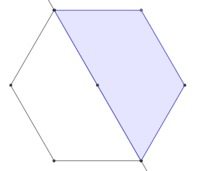each one is a trapezoid and a reflection across the line takes one trapezoid to the other.

2. There are many ways to construct interesting partitions. Any line through the center of the hexagon (which is at the intersection of any two diagonals) divides the hexagon into two congruent pieces.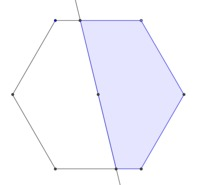A 180o rotation about the center shows this congruence because the line will go to itself and each vertex will be mapped to the vertex opposite it. Since line segments are taken to line segments, all the vertices and sides of the two halves will "match up" under this rotation, showing they are congruent.

Or start with a line through the center, and "cut out" any piece of one of the halves and "add it back in" by rotating it 180o about the center of the hexagon. Here are three examples: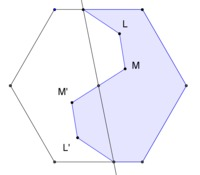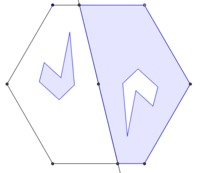In each case, the figures were constructed so that a 180o rotation about the center will "line them up, one on top of the other."

3. Here is one way: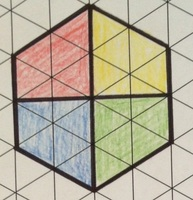We know they are congruent because if we consider the two lines shown below: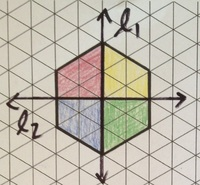The yellow region is the image of the red region when we reflect across $\ell_1$, so those two regions are congruent. The green region is the image of the blue region when we reflect across $\ell_1$, so those two regions are congruent. The yellow region is the image of the green region when we reflect across $\ell_2$, so those two regions are congruent. So all four regions are congruent.

4. Here is one way:We can show they are congruent using reflections about the two lines shown below:All four regions on the left side of $\ell_1$ are congruent to their mirror images on the right. We can show the purple and orange regions are congruent by reflecting across $\ell_2$. We can get the rest of the pair-wise congruencies by rotating a multiple of 60 degrees around point $P$. So all eight regions are congruent.

Solution: Solutions to the bonus questions

Bonus 1: Here is one way:Bonus 2: Here is one way:Note that the regions don't need to be bounded by simple closed curves.

Bonus 3: Here is one way: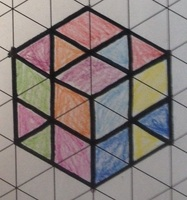Note that the regions don't need to be bounded by simple closed curves.